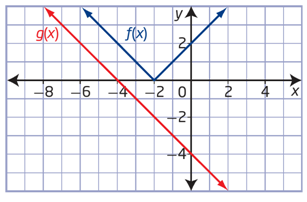# Composite functions#### Everything You Need in One Place

Homework problems? Exam preparation? Trying to grasp a concept or just brushing up the basics? Our extensive help & practice library have got you covered.#### Learn and Practice With Ease

Our proven video lessons ease you through problems quickly, and you get tonnes of friendly practice on questions that trip students up on tests and finals.#### Instant and Unlimited Help

Our personalized learning platform enables you to instantly find the exact walkthrough to your specific type of question. Activate unlimited help now!

0/2
##### Intros
###### Lessons
1. What is a "Composite Function"?
2. Quick review on basic operations with functions.
3. Composition of Functions: putting one function inside another function!
0/18
##### Examples
###### Lessons
1. Introduction to Composite Functions
If $f(x)= 4x-5$
$g(x)=8x^2+6$
Determine
1. $(f\circ g)(x)$
2. $(g\circ f)(x)$
3. $(f\circ f)(x)$
4. $(g\circ g)(x)$
5. $(f\circ g)(2)$
evaluate in two different ways
2. Evaluate a Composite Function Graphically
Use the graphs of $f(x)$ and $g(x)$ to evaluate the following:1. $f(g(-4))$
2. $f(g(0))$
3. $g(f(-2))$
4. $g(f(-3))$
3. Determine the Composition of Three Functions
Use the functions $f(x)=3x,$
$g(x)=x-7$
and $h(x)=x^2$
to determine each of the following:
1. $(f\circ g\circ h)(x)$
2. $g(f(h(x)))$
3. $f(h(g(x)))$
4. $(h\circ g\circ f)(x)$
4. Determine the Original Functions From a Composite Function
If $h(x)=(f\circ g)(x)$
determine $f(x)$
and $g(x)$
1. $h(x)=(7x-5)^3-4(7x-5)+1$
2. $h(x)=\sqrt{4x^3-9}$, give two possible sets of solutions
5. Composite Functions with Restrictions
Consider $f(x)=\sqrt{x-13}$
and $g(x)=x^2+4$, for each of the function below:
1. Determine:
i)
$(f\circ g)(x)$
ii)
$(g\circ f)(x)$
2. State the domain and range of:
i)
$f(x)$
ii)
$g(x)$
iii) $(f\circ g)(x)$
iv)
$(g\circ f)(x)$
3. Sketch the graph of:
i)
$f(x)$
ii)
$g(x)$
iii) $(f\circ g)(x)$
iv)
$(g\circ f)(x)$
6. Composite Functions with Restrictions
Consider $f(x)=\sqrt{x-13}$
and $g(x)=x^2+4$, for each of the function below:
i) state the domain and range
ii) sketch the graph.
1. $f(x)$
2. $g(x)$
3. $(f\circ g)(x)$